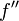# Newton's method for optimization of a function of one variable

## Definition

Newton's method for optimization of a function of one variable is a method obtained by slightly tweaking Newton's method for root-finding for a function of one variable to find the points of local extrema (maxima and minima) for a differentiable function with known derivative.

The key insight is that point of local extremum implies critical point, so that in order to find the points of local extrema for the function, it suffices to find the critical points. With the differentiability assumption, this means that it suffices to find the points where the derivative is zero.

In the case that we are interested in finding local extrema of a particular type only (for instance, only local maxima or only local minima) we can impose further restrictions on the nature of our search so as to avoid converging to local extrema of the other kind. These restrictions can be seen as arising from the first derivative test and/or second derivative test.

## Iterative step

The initial guess$x_0$ for the point of local extremum needs to be supplied to initiate the method.

For any nonnegative integer$k$, define:$x_{k+1} := x_k - \frac{f'(x_k)}{f''(x_k)}$

This is obtained from Newton's method for root-finding for a function of one variable applied to the function$f'$.

Because of the appearance of$f''$ in the denominator at each iterative step, Newton's method for optimization is termed a second-order method. This becomes more relevant for the multidimensional version (Newton's method for optimization of a function of multiple variables) because in that case, the number of numerical entries describing a derivative is exponential in the order of derivative.

Since the iterative step as described here is simply attempting to converge to a root, it could be converging towards a local extremum of the wrong type. Fortunately, before making the step, we can test if we seem to be headed towards the desirable type of extremum. Explicitly, this follows from the second derivative test: if we are close enough to the point of local extremum, then a positive second derivative signifies a local minimum, whereas a negative second derivative signifies a local maximum.

What if we're at a point that has a wrong sign of second derivative? There are three options:

• Apply a few iterations and see if one gets to a point with the correct sign of second derivative.
• Simply choose a new starting point.
• Apply enough iterations to converge to the corresponding root of the derivative, then factor out that root from the derivative to obtain a new function that we then find a root for. This approach is most helpful for polynomials where finding one factor allows us to reduce the degree of the polynomial and therefore find other factors with more ease.

## Cautionary note about results for Newton's method

Newton's method as an optimization procedure relies on Newton's method as a root-finding algorithm applied to the derivative. In particular, this means that all the facts about the behavior of Newton's method as a root-finding algorithm, when translated to Newton's method in optimization, need to be adjusted by replacing the function by its derivative. Some examples are below.

• Newton's method as a root-finding algorithm converges in one step if the function is linear. Therefore, Newton's method for optimization converges in one step if the function is quadratic.
• Newton's method as a root-finding algorithm converges quadratically when close to the point, assuming that the second derivative is nonzero at and around the point (plus some other assumptions?). When translated to Newton's method for optimization, this is a statement about the third derivative.